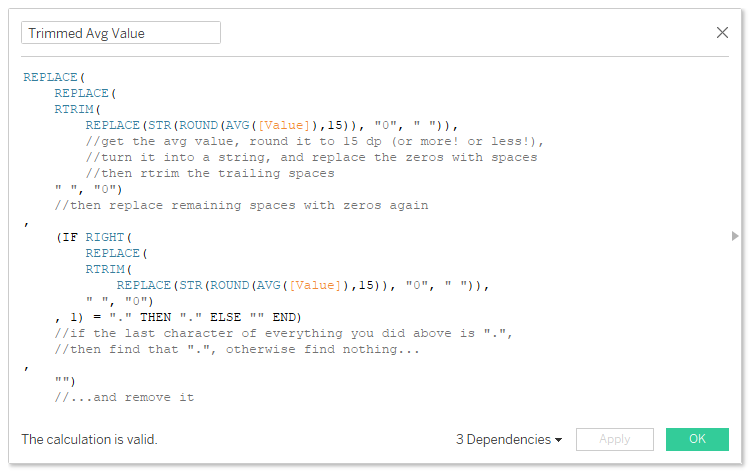## Strings and Roundabouts, pt.2: dynamic decimals

Quite a while ago, I wrote what I thought was a highly-specific blog for a niche use-case – dynamically rounding your Tableau numbers to millions, thousands, billions, or whatever made sense. That ended up being one of my most-viewed blogs.

So today, I’m writing a follow-up. How do you round the number of decimals to a number that actually makes sense?

Take this input data:

If you plot this in Tableau, it’s normally enough to set the default format to Number (standard). That gives us this:

But if you don’t like the scientific formatting for Thing 2 and 7 in Type b, you’ll have to set the number of decimal places to the right number. But that’ll give you this:

Ew.

You can get around this with strings. I don’t use this too often, but it comes in handy now and again. Here’s the formula that you can copy/paste and use in your own workbooks:

`REPLACE( REPLACE( RTRIM( REPLACE(STR(ROUND(AVG([Value]),15)), "0", " ")), //get the avg value, round it to 15 dp (or more! or less!), //turn it into a string, and replace the zeros with spaces //then rtrim the trailing spaces " ", "0") //then replace remaining spaces with zeros again , (IF RIGHT( REPLACE( RTRIM( REPLACE(STR(ROUND(AVG([Value]),15)), "0", " ")), " ", "0") , 1) = "." THEN "." ELSE "" END) //if the last character of everything you did above is ".", //then find that ".", otherwise find nothing… , "") //…and remove it`

Working from inside out, the calculation does this:

1. Take the AVG() of your field. You’ll want to change this to whichever aggregation makes most sense for your use case.
e.g. 6.105
2. Rounds that aggregation to 15 decimal places. This is almost definitely going to be enough, but hey, you might need to up it to 20 or so. I have never needed to do this.
e.g. 6.105000000000000
3. Turns that into a string.
e.g. “6.105000000000000”
4. Replaces the zeros in the string to spaces.
e.g. “6.1 5 ”
5. Uses RTRIM() to remove all trailing spaces on the right of the string.
e.g. “6.1 5”
6. Replaces any remaining spaces with zeros again.
e.g. “6.105”
7. If the last character of the string is a decimal point, then there are no decimals needed, so it removes that decimal point by replacing it with nothing; otherwise, it leaves it where it is.
e.g. “6.105”

And there you go – the number is formatted as a string to the exact number of decimals you’ve got in your Excel file.

Interestingly, there are some differences between the way REPLACE() and REGEX_REPLACE() work. It seems that REPLACE() will wait for the aggregation, rounding, and conversion to string before doing anything, whereas REGEX_REPLACE() will give you the same issues you get as if you just turn a number straight into a string without rounding first.

You can see all this in a workbook on Tableau Public here: https://public.tableau.com/profile/gwilym#!/vizhome/200501stringsandroundaboutspt2/Examples

London, UK